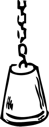1 Propositional Logic
(propositional_logic_primer.pptx) propositional_logic.pdf (2x3)
propositional-sat.ppt
propositional-bdds.ppt
Paper on MiniSat, MiniSat
SAT-Race 2006
EduSAT - a basic and small SAT solver for educational purposes, and corresponding EduSAT project proposals.
Bryant's paper on BDDs
CUDD (Documentation)
miniBDD
2 First-order theories
First-order theories primer.ppt
3 Equalities and Uninterpreted Functions
uf.pdf (2x3)
4 Decision Procedures for Equality Logic and Uninterpreted Functions
equality-intro.ppt
equality-transitivity-constraints.ppt
equality-range-allocation.ppt
equality-e-graphs.ppt
The small model property: How small can it be?
Boolean satisfiability with transitivity constraints
5 Linear Arithmetic
linear-simplex.ppt
linear-fourier_motzkin.pdf (2x3)
linear-branch-and-bound.ppt
linear-gomory.pdf
linear-omega.pdf (2x3)
Dutertre, de Moura: A Fast Linear-Arithmetic Solver for DPLL(T)
Pugh: The Omega test
6 Bit Vectors
bit-vectors.pdf (2x3)
7 Arrays